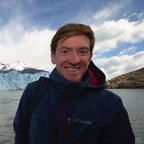# Linking particle positions to form trajectories

First published:

Last Edited:

Number of edits:

Nanoparticle tracking analysis relies on being able to form tracks of individual particles. After locating particles for nanoparticle tracking and refining the position of nanoparticles on an image, we must link the position of particles from one frame to the next. frame the problem as an optimization problem. First, one must calculate the probability of a particle diffusing a given distance (in the plane) in a given time:

$$P(\delta|\tau) = \frac{1}{4\pi D\tau}\exp\left(-\frac{\delta^2}{4D\tau}\right)$$

For N noninteracting, identical particles, the probability is the product of probabilities:

$$P({\delta_i}|\tau) = \left(\frac{1}{4\pi D\tau}\right)^N \exp\left(-\sum_{i=1}^{N}\frac{\delta_i^2}{4D\tau}\right)$$

Therefore, the most likely assignment of particles from one frame to the next is the one that maximizes

$$P$$
, or equivalently, the one that minimizes
$$\sum\delta_i$$

For N particles, the number of pairs to calculate

$$P$$
is
$$N!$$
which makes it computationally impractical for large numbers of particles. The most straightforward approach is to limit the computation of the distance to only particles within a certain radius
$$L$$
, which is equivalent to truncating at
$$\delta=L$$
. If samples are diluted enough it is likely that this will mean, probably, only one possible particle assignment from one frame to the next.

In they also identify the possibility of having sub-networks of possible particles link to each other from one frame to another. Therefore the optimization problem gets a reduced dimension, to only optimizations within sub networks. The algorithm's complexity is

$$O(N\ln N)$$

However, the open question is what happens if on one frame there are N particles and in the following there are M particles located (or, more than in one frame, in one sub-network). They suggest in those cases, the particles which are not linked from one frame to the other are kept in memory in case they re-appear some frames later, and they get assigned

$$\delta_i=L$$
.

The authors of the paper also suggest that this linking procedure works well enough provided that particle separation is larger than the typical displacement at each time step. Therefore, the optimal cutoff parameter should be in the range:

$$\delta \lt L \lt a/2$$